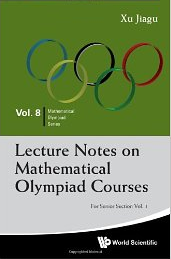## Lecture Notes on Mathematical Olympiad Courses

### Xu JiaguThe two books by Xu Jiagu constitute Volume 8 in the Mathematical Olympiad Series published by the World Scientific. Each of the books contains 15 chapters, called lectures - I may only guess why. Chapters open with a short introduction (notations, important notions, basic theorems), followed by several examples, with detailed solutions, and lists A and B of practice problems to be solved independently. Solutions to all the problems in both lists appear at the end of the book.

Some of the example problems, most from List A, and practically all problems from List B have been plucked from various national (with emphasis on China's) mathematical competitions and the IMO. There is noticeable correlation between sample problems and those in List A, such that the latter are clearly intended for practicing techniques the author discusses in the sample problems. Problems in List B require more experience and originality. It is obvious that the author went to a considerable length gathering and organizing the problems according to the solution techniques and difficulty.

Of the problem books I own, the two books at hand resemble most Arthur Engel's Problem Solving Strategies in structure and probably intention. In contrast, Xu Jiagu's explanations are more frugal, and only few problems come with more than one solution. The brevity of introductory explanations is made up by the careful selection into List A problems closely related to the samples solved by the author. Consistent with this approach, Xu Jiagu's topics are more narrowly defined, making for more chapters, each better focused.

The books were in my possession for a week now. While browsing I found only one insignificant typo; of the several problems I have solved, I can honestly claim that for only one problem my solution was better - clearer and based on more fundamental skills - than the one in the book. With this experience, I see the books used beneficially by olympiad coaches and, individually, by aspiring olympians. Many problems, especially among the introductory samples, could be used in math circles or as extras in honors classes.

#### Content of Volume 1

• Fractional Equations
• Higher Degree Polynomial Equations
• Irrational Equations
• Indicial Functions and Logarithmic Functions
• Trigonometric Functions
• Law of Sines and Law of Cosines
• Manipulations of Trigonometric Expressions
• Extreme Values of Functions and Mean Inequality
• Extreme Value Problems in Trigonometry
• Fundamental Properties of Circles
• Relation of Line and Circle and Relation of Circles
• Cyclic Polygons
• Power of a Point with Respect to a Circle
• Some Important Theorems in Geometry
• Five Centers of a Triangle

#### Content of Volume 2

• Mathematical Induction
• Arithmetic Progressions and Geometric Progressions
• Recursive Sequences
• Summation of Various Sequences
• Some Fundamental Theorems on Congruence
• Chinese Remainder Theorem and Order of Integer
• Diophantine Equations (III)
• Pythagorean Triples and Pell's Equations
• Some Important Inequalities(I)
• Some Important Inequalities(II)
• Some Methods for Solving Inequalities
• Some Basic Methods in Counting(I)
• Some Basic Methods in Counting(II)
• Introduction to Functional EquationsLecture Notes on Mathematical Olympiad Courses for Senior Section, by Xu Jiagu. Two volumes. World Scientific. Softcover (2012), 260 pp, \$73.50. ISBN 978-9814368940